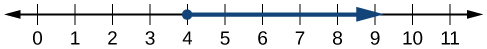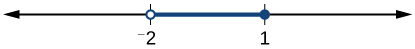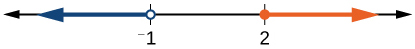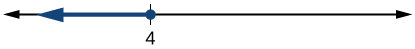## Linear Inequalities and Absolute Value Inequalities

### Learning Objectives

In this section you will:

• Use interval notation.
• Use properties of inequalities.
• Solve inequalities in one variable algebraically.
• Solve absolute value inequalities.Figure 1.

It is not easy to make the honor role at most top universities. Suppose students were required to carry a course load of at least 12 credit hours and maintain a grade point average of 3.5 or above. How could these honor roll requirements be expressed mathematically? In this section, we will explore various ways to express different sets of numbers, inequalities, and absolute value inequalities.

### Using Interval Notation

Indicating the solution to an inequality such as$\,x\ge 4\,$can be achieved in several ways.

We can use a number line as shown in (Figure). The blue ray begins at$\,x=4\,$and, as indicated by the arrowhead, continues to infinity, which illustrates that the solution set includes all real numbers greater than or equal to 4.Figure 2.

We can use set-builder notation:$\,\left\{x|x\ge 4\right\},$which translates to “all real numbers x such that x is greater than or equal to 4.” Notice that braces are used to indicate a set.

The third method is interval notation, in which solution sets are indicated with parentheses or brackets. The solutions to$\,x\ge 4\,$are represented as$\,\left[4,\infty \right).\,$This is perhaps the most useful method, as it applies to concepts studied later in this course and to other higher-level math courses.

The main concept to remember is that parentheses represent solutions greater or less than the number, and brackets represent solutions that are greater than or equal to or less than or equal to the number. Use parentheses to represent infinity or negative infinity, since positive and negative infinity are not numbers in the usual sense of the word and, therefore, cannot be “equaled.” A few examples of an interval, or a set of numbers in which a solution falls, are$\,\left[-2,6\right),$or all numbers between$\,-2\,$and$\,6,$including$\,-2,$but not including$\,6;$$\left(-1,0\right),$all real numbers between, but not including$\,-1\,$and$\,0;$and$\,\left(-\infty ,1\right],$all real numbers less than and including$\,1.\,$(Figure) outlines the possibilities.

Set Indicated Set-Builder Notation Interval Notation
All real numbers between a and b, but not including a or b $\left\{x|a<x<b\right\}$ $\left(a,b\right)$
All real numbers greater than a, but not including a $\left\{x|x>a\right\}$ $\left(a,\infty \right)$
All real numbers less than b, but not including b $\left\{x|x<b\right\}$ $\left(-\infty ,b\right)$
All real numbers greater than a, including a $\left\{x|x\ge a\right\}$ $\left[a,\infty \right)$
All real numbers less than b, including b $\left\{x|x\le b\right\}$ $\left(-\infty ,b\right]$
All real numbers between a and b, including a $\left\{x|a\le x<b\right\}$ $\left[a,b\right)$
All real numbers between a and b, including b $\left\{x|a<x\le b\right\}$ $\left(a,b\right]$
All real numbers between a and b, including a and b $\left\{x|a\le x\le b\right\}$ $\left[a,b\right]$
All real numbers less than a or greater than b $\left\{x|x<a\,\text{or}\,x>b\right\}$ $\left(-\infty ,a\right)\cup \left(b,\infty \right)$
All real numbers $\left\{x|x\text{ is all real numbers}\right\}$ $\left(-\infty ,\infty \right)$

### Using Interval Notation to Express All Real Numbers Greater Than or Equal to a

Use interval notation to indicate all real numbers greater than or equal to$\,-2.$

### Try It

Use interval notation to indicate all real numbers between and including$\,-3\,$and$\,5.$

### Using Interval Notation to Express All Real Numbers Less Than or Equal to a or Greater Than or Equal to b

Write the interval expressing all real numbers less than or equal to$\,-1\,$or greater than or equal to$\,1.$

### Try It

Express all real numbers less than$\,-2\,$or greater than or equal to 3 in interval notation.

### Using the Properties of Inequalities

When we work with inequalities, we can usually treat them similarly to but not exactly as we treat equalities. We can use the addition property and the multiplication property to help us solve them. The one exception is when we multiply or divide by a negative number; doing so reverses the inequality symbol.

### Properties of Inequalities

$\begin{array}{ll}Addition\text{ }Property\hfill & \phantom{\rule{2em}{0ex}}\text{If }a<b,\text{ then }a+c<b+c.\hfill \\ \hfill & \hfill \\ Multiplication\text{ }Property\hfill & \phantom{\rule{2em}{0ex}}\text{If }a<b\text{ and }c>0,\text{ then }ac<bc.\hfill \\ \hfill & \phantom{\rule{2em}{0ex}}\text{If }a<b\text{ and }c<0,\text{ then }ac>bc.\hfill \end{array}$

These properties also apply to$\,a\le b,$$a>b,$and$\,a\ge b.$

Illustrate the addition property for inequalities by solving each of the following:

• (a) $x-15<4$
• (b) $6\ge x-1$
• (c) $x+7>9$

### Try It

Solve:$\,3x-2<1.$

### Demonstrating the Multiplication Property

Illustrate the multiplication property for inequalities by solving each of the following:

1. $3x<6$
2. $-2x-1\ge 5$
3. $5-x>10$

### Try It

Solve:$\,4x+7\ge 2x-3.$

### Solving Inequalities in One Variable Algebraically

As the examples have shown, we can perform the same operations on both sides of an inequality, just as we do with equations; we combine like terms and perform operations. To solve, we isolate the variable.

### Solving an Inequality Algebraically

Solve the inequality:$\,13-7x\ge 10x-4.$

### Try It

Solve the inequality and write the answer using interval notation:$\,-x+4<\frac{1}{2}x+1.$

### Solving an Inequality with Fractions

Solve the following inequality and write the answer in interval notation:$\,-\frac{3}{4}x\ge -\frac{5}{8}+\frac{2}{3}x.$

### Try It

Solve the inequality and write the answer in interval notation:$\,-\frac{5}{6}x\le \frac{3}{4}+\frac{8}{3}x.$

### Understanding Compound Inequalities

A compound inequality includes two inequalities in one statement. A statement such as$\,4<x\le 6\,$means$\,4<x\,$and$\,x\le 6.\,$There are two ways to solve compound inequalities: separating them into two separate inequalities or leaving the compound inequality intact and performing operations on all three parts at the same time. We will illustrate both methods.

### Solving a Compound Inequality

Solve the compound inequality:$\,3\le 2x+2<6.$

### Try It

Solve the compound inequality:$\,4<2x-8\le 10.$

### Solving a Compound Inequality with the Variable in All Three Parts

Solve the compound inequality with variables in all three parts:$\,3+x>7x-2>5x-10.$

### Try It

Solve the compound inequality:$\,3y<4-5y<5+3y.$

### Solving Absolute Value Inequalities

As we know, the absolute value of a quantity is a positive number or zero. From the origin, a point located at$\,\left(-x,0\right)\,$has an absolute value of$\,x,$as it is x units away. Consider absolute value as the distance from one point to another point. Regardless of direction, positive or negative, the distance between the two points is represented as a positive number or zero.

An absolute value inequality is an equation of the form

$|A|<B,\,|A|\le B,\,\,|A|>B,\,\text{or }\,\,|A|\ge B,$

Where A, and sometimes B, represents an algebraic expression dependent on a variable x. Solving the inequality means finding the set of all $\,x$values that satisfy the problem. Usually this set will be an interval or the union of two intervals and will include a range of values.

There are two basic approaches to solving absolute value inequalities: graphical and algebraic. The advantage of the graphical approach is we can read the solution by interpreting the graphs of two equations. The advantage of the algebraic approach is that solutions are exact, as precise solutions are sometimes difficult to read from a graph.

Suppose we want to know all possible returns on an investment if we could earn some amount of money within $200 of$600. We can solve algebraically for the set of x-values such that the distance between$\,x\,$and 600 is less than 200. We represent the distance between$\,x\,$and 600 as$\,|x-600|,$and therefore,$\,|x-600|\le 200\,$or

$\begin{array}{c}-200\le x-600\le 200\\ -200+600\le x-600+600\le 200+600\\ 400\le x\le 800\end{array}$

This means our returns would be between $400 and$800.

To solve absolute value inequalities, just as with absolute value equations, we write two inequalities and then solve them independently.

### Absolute Value Inequalities

For an algebraic expression X, and$\,k>0,$an absolute value inequality is an inequality of the form

$\begin{array}{l}|X|<k\text{ is equivalent to }-k<X<k\hfill \\ |X|>k\text{ is equivalent to }X<-k\text{ or }X>k\hfill \end{array}$

These statements also apply to$\,|X|\le k\,$and$\,|X|\ge k.$

### Determining a Number within a Prescribed Distance

Describe all values$\,x\,$within a distance of 4 from the number 5.

### Try It

Describe all x-values within a distance of 3 from the number 2.

### Solving an Absolute Value Inequality

Solve $|x-1|\le 3$.

### Using a Graphical Approach to Solve Absolute Value Inequalities

Given the equation $y=-\frac{1}{2}|4x-5|+3,$determine the x-values for which the y-values are negative.

### Try It

Solve$\,-2|k-4|\le -6.$

Access these online resources for additional instruction and practice with linear inequalities and absolute value inequalities.

### Key Concepts

• Interval notation is a method to indicate the solution set to an inequality. Highly applicable in calculus, it is a system of parentheses and brackets that indicate what numbers are included in a set and whether the endpoints are included as well. See (Figure) and (Figure).
• Solving inequalities is similar to solving equations. The same algebraic rules apply, except for one: multiplying or dividing by a negative number reverses the inequality. See (Figure), (Figure), (Figure), and (Figure).
• Compound inequalities often have three parts and can be rewritten as two independent inequalities. Solutions are given by boundary values, which are indicated as a beginning boundary or an ending boundary in the solutions to the two inequalities. See (Figure) and (Figure).
• Absolute value inequalities will produce two solution sets due to the nature of absolute value. We solve by writing two equations: one equal to a positive value and one equal to a negative value. See (Figure) and (Figure).
• Absolute value inequalities can also be solved by graphing. At least we can check the algebraic solutions by graphing, as we cannot depend on a visual for a precise solution. See (Figure).

### Section Exercises

#### Verbal

When solving an inequality, explain what happened from Step 1 to Step 2:

$\begin{array}{ll}\text{Step 1}\hfill & \phantom{\rule{2em}{0ex}}-2x>6\hfill \\ \text{Step 2}\hfill & \phantom{\rule{3em}{0ex}}x<-3\hfill \end{array}$

When solving an inequality, we arrive at:

$\begin{array}{l}x+2<x+3\hfill \\ \phantom{\rule{1.2em}{0ex}}2<3\hfill \end{array}$

Explain what our solution set is.

When writing our solution in interval notation, how do we represent all the real numbers?

When solving an inequality, we arrive at:

$\begin{array}{l}x+2>x+3\hfill \\ \phantom{\rule{1.2em}{0ex}}2>3\hfill \end{array}$

Explain what our solution set is.

Describe how to graph$\,y=|x-3|$

#### Algebraic

For the following exercises, solve the inequality. Write your final answer in interval notation.

$4x-7\le 9$

$3x+2\ge 7x-1$

$-2x+3>x-5$

$4\left(x+3\right)\ge 2x-1$

$-\frac{1}{2}x\le \frac{-5}{4}+\frac{2}{5}x$

$-5\left(x-1\right)+3>3x-4-4x$

$-3\left(2x+1\right)>-2\left(x+4\right)$

$\frac{x+3}{8}-\frac{x+5}{5}\ge \frac{3}{10}$

$\frac{x-1}{3}+\frac{x+2}{5}\le \frac{3}{5}$

For the following exercises, solve the inequality involving absolute value. Write your final answer in interval notation.

$|x+9|\ge -6$

$|2x+3|<7$

$|3x-1|>11$

$|2x+1|+1\le 6$

$|x-2|+4\ge 10$

$|-2x+7|\le 13$

$|x-7|<-4$

$|x-20|>-1$

$|\frac{x-3}{4}|<2$

For the following exercises, describe all the x-values within or including a distance of the given values.

Distance of 5 units from the number 7

Distance of 3 units from the number 9

Distance of10 units from the number 4

Distance of 11 units from the number 1

$-4<3x+2\le 18$

$3x+1>2x-5>x-7$

$3y<5-2y<7+y$

$2x-5<-11\text{ or }5x+1\ge 6$

$x+7<x+2$

#### Graphical

For the following exercises, graph the function. Observe the points of intersection and shade the x-axis representing the solution set to the inequality. Show your graph and write your final answer in interval notation.

$|x-1|>2$

$|x+3|\ge 5$

$|x+7|\le 4$

$|x-2|<7$

$|x-2|<0$

For the following exercises, graph both straight lines (left-hand side being y1 and right-hand side being y2) on the same axes. Find the point of intersection and solve the inequality by observing where it is true comparing the y-values of the lines.

$x+3<3x-4$

$x-2>2x+1$

$x+1>x+4$

$\frac{1}{2}x+1>\frac{1}{2}x-5$

$4x+1<\frac{1}{2}x+3$

#### Numeric

For the following exercises, write the set in interval notation.

$\left\{x|-1<x<3\right\}$

$\left\{x|x\ge 7\right\}$

$\left\{x|x<4\right\}$

$\left\{\,x|\,x\text{ is all real numbers}\right\}$

For the following exercises, write the interval in set-builder notation.

$\left(-\infty ,6\right)$

$\left(4,+\infty \right)$

$\left[-3,5\right)$

$\left[-4,1\right]\cup \left[9,\infty \right)$

For the following exercises, write the set of numbers represented on the number line in interval notation.#### Technology

For the following exercises, input the left-hand side of the inequality as a Y1 graph in your graphing utility. Enter y2 = the right-hand side. Entering the absolute value of an expression is found in the MATH menu, Num, 1:abs(. Find the points of intersection, recall (2nd CALC 5:intersection, 1st curve, enter, 2nd curve, enter, guess, enter). Copy a sketch of the graph and shade the x-axis for your solution set to the inequality. Write final answers in interval notation.

$|x+2|-5<2$

$\frac{-1}{2}|x+2|<4$

$|4x+1|-3>2$

$|x-4|<3$

$|x+2|\ge 5$

#### Extensions

Solve$\,|3x+1|=|2x+3|$

Solve${x}^{2}-x>12$

$\frac{x-5}{x+7}\le 0,$$x\ne -7$

$p=-{x}^{2}+130x-3000\,$is a profit formula for a small business. Find the set of x-values that will keep this profit positive.

#### Real-World Applications

In chemistry the volume for a certain gas is given by$\,V=20T,$where V is measured in cc and T is temperature in ºC. If the temperature varies between 80ºC and 120ºC, find the set of volume values.

#### Complex Numbers

For the following exercises, use the quadratic equation to solve.

${x}^{2}-5x+9=0$

$2{x}^{2}+3x+7=0$

For the following exercises, name the horizontal component and the vertical component.

$4-3i$

$-2-i$

For the following exercises, perform the operations indicated.

$\left(9-i\right)-\left(4-7i\right)$

$\left(2+3i\right)-\left(-5-8i\right)$

$2\sqrt{-75}+3\sqrt{25}$

$\sqrt{-16}+4\sqrt{-9}$

$-6i\left(i-5\right)$

${\left(3-5i\right)}^{2}$

$\sqrt{-4}·\sqrt{-12}$

$\sqrt{-2}\left(\sqrt{-8}-\sqrt{5}\right)$

$\frac{2}{5-3i}$

$\frac{3+7i}{i}$

For the following exercises, solve the quadratic equation by factoring.

$2{x}^{2}-7x-4=0$

$3{x}^{2}+18x+15=0$

$25{x}^{2}-9=0$

$7{x}^{2}-9x=0$

For the following exercises, solve the quadratic equation by using the square-root property.

${x}^{2}=49$

${\left(x-4\right)}^{2}=36$

For the following exercises, solve the quadratic equation by completing the square.

${x}^{2}+8x-5=0$

$4{x}^{2}+2x-1=0$

For the following exercises, solve the quadratic equation by using the quadratic formula. If the solutions are not real, state No real solution.

$2{x}^{2}-5x+1=0$

$15{x}^{2}-x-2=0$

For the following exercises, solve the quadratic equation by the method of your choice.

${\left(x-2\right)}^{2}=16$

${x}^{2}=10x+3$

#### Other Types of Equations

For the following exercises, solve the equations.

${x}^{\frac{3}{2}}=27$

${x}^{\frac{1}{2}}-4{x}^{\frac{1}{4}}=0$

$4{x}^{3}+8{x}^{2}-9x-18=0$

$3{x}^{5}-6{x}^{3}=0$

$\sqrt{x+9}=x-3$

$\sqrt{3x+7}+\sqrt{x+2}=1$

$|3x-7|=5$

$|2x+3|-5=9$

#### Linear Inequalities and Absolute Value Inequalities

For the following exercises, solve the inequality. Write your final answer in interval notation.

$5x-8\le 12$

$-2x+5>x-7$

$\frac{x-1}{3}+\frac{x+2}{5}\le \frac{3}{5}$

$|3x+2|+1\le 9$

$|5x-1|>14$

$|x-3|<-4$

For the following exercises, solve the compound inequality. Write your answer in interval notation.

$-4<3x+2\le 18$

$3y<1-2y<5+y$

For the following exercises, graph as described.

Graph the absolute value function and graph the constant function. Observe the points of intersection and shade the x-axis representing the solution set to the inequality. Show your graph and write your final answer in interval notation.

$|x+3|\ge 5$

Graph both straight lines (left-hand side being y1 and right-hand side being y2) on the same axes. Find the point of intersection and solve the inequality by observing where it is true comparing the y-values of the lines. See the interval where the inequality is true.

$x+3<3x-4$

### Chapter Practice Test

Graph the following:$\,2y=3x+4.$

Find the x- and y-intercepts for the following:

Find the x- and y-intercepts of this equation, and sketch the graph of the line using just the intercepts plotted.

$3x-4y=12$

Find the exact distance between$\,\left(5,-3\right)\,$and$\,\left(-2,8\right).\,$Find the coordinates of the midpoint of the line segment joining the two points.

Write the interval notation for the set of numbers represented by$\,\left\{x|x\le 9\right\}.$

Solve for x:$\,5x+8=3x-10.$

Solve for x:$\,3\left(2x-5\right)-3\left(x-7\right)=2x-9.$

Solve for x:$\,\frac{x}{2}+1=\frac{4}{x}$

Solve for x:$\,\frac{5}{x+4}=4+\frac{3}{x-2}.$

The perimeter of a triangle is 30 in. The longest side is 2 less than 3 times the shortest side and the other side is 2 more than twice the shortest side. Find the length of each side.

$\frac{{x}^{2}}{3}-x=\frac{-1}{2}$

Solve:$\,3x-8\le 4.$

Solve:$\,|2x+3|<5.$

Solve:$\,|3x-2|\ge 4.$

For the following exercises, find the equation of the line with the given information.

Passes through the points$\,\left(-4,2\right)\,$and$\,\left(5,-3\right).$

Has an undefined slope and passes through the point$\,\left(4,3\right).$

Passes through the point$\,\left(2,1\right)\,$and is perpendicular to$\,y=\frac{-2}{5}x+3.$

Add these complex numbers:$\,\left(3-2i\right)+\left(4-i\right).$

Simplify:$\,\sqrt{-4}+3\sqrt{-16}.$

Multiply:$\,5i\left(5-3i\right).$

Divide:$\,\frac{4-i}{2+3i}.$

Solve this quadratic equation and write the two complex roots in$\,a+bi\,$form:$\,{x}^{2}-4x+7=0.$

Solve:$\,{\left(3x-1\right)}^{2}-1=24.$

Solve:$\,{x}^{2}-6x=13.$

Solve:$\,4{x}^{2}-4x-1=0$

Solve:

$\sqrt{x-7}=x-7$

Solve:$\,2+\sqrt{12-2x}=x$

Solve:$\,{\left(x-1\right)}^{\frac{2}{3}}=9$

For the following exercises, find the real solutions of each equation by factoring.

$2{x}^{3}-{x}^{2}-8x+4=0$

${\left(x+5\right)}^{2}-3\left(x+5\right)-4=0$

### Glossary

compound inequality
a problem or a statement that includes two inequalities
interval
an interval describes a set of numbers within which a solution falls
interval notation
a mathematical statement that describes a solution set and uses parentheses or brackets to indicate where an interval begins and ends
linear inequality
similar to a linear equation except that the solutions will include sets of numbers